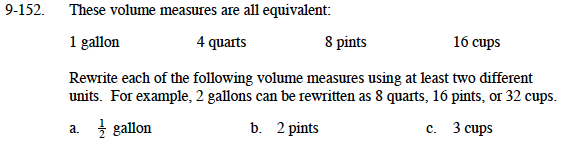### Home > MC1 > Chapter 9 > Lesson 9.3.3 > Problem9-152

9-152.$\frac{4\text{ quarts}}{1\text{ gallon}}=\frac{x\text{ quarts}}{\frac{1}{2}\text{ gallon}}$

x = 2 quarts

2 pints is a quarter of 8 pints, or a quarter of one gallon. How many quarts is that? How many cups?

You can set up proportions as shown in part (a) or use logic, as shown in part (b). Which way do you prefer?